+ +

# Collection of the wave front

The collection of the wave front presides over the conduct of a review aberrometrique. The optical quality of the eye is very correlated with the existence of phase shifts (optical path differences) within the wave front. Applications of wave-front collection mainly the study of Visual symptoms, suspected the origin to be optical. In refractive surgery, preoperative study of optical aberrations will determine the value of a personalized treatment and program delivery by guiding the laser shots.

### Analysis of the Wavefront in OPD

The analysis of the optical aberrations of the eye is based on the works of Hartmann and Tscherning-19e century, which gave rise to two main categories of wave front analyzers.

Hartmann laid the Foundation of the objective of the wave front collected after reflection foveolaire and analysis crossing of the different areas of the eye (vitreous, lens, anterior Chamber, posterior, stroma and anterior surface of the cornea, tear film) ("outgoing reflective aberrometry" Hartmann-Shack type wave front analyzers) (3).
Conversely, other systems analyze the incident wave front. Type Analyzer Tscherning studied the wave front through the distortion of a focus on the retina. Other systems are based on a similar principle, but with a sequential collection and study of the displacement of the projection of beams laser compared to the reference beam (Laser Ray Tracing), or after skiascopie automated by a rotating slot (2). Finally, some devices allow the assessment of the wave front by seeking the subjective answers of the patient subject to the projection of light rays on the retina.
All these systems are governed around a common principle: the reconstitution of the wave as a three-dimensional surface front is made from the analysis of the distortion suffered by a signal. Hartmann Schack being the most frequent type systems, they will serve as examples.

### Study of the reflected wave front: Hartmann-Shack systems

They derive from the principle of the disc of Scheiner (philosopher and astronomer at the beginning of the 17e (century), that allows to reveal the parasite image of a point seen by an eye equipped with optical aberrations. The technique has evolved and has been perfected through successive works of Hartmann and Schack (3).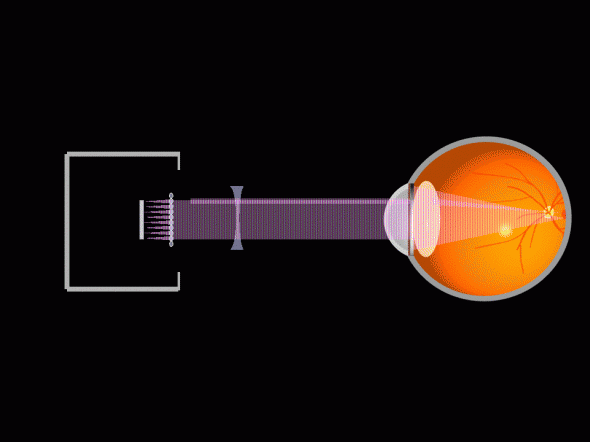#### Principles of the code of the wave front

Schematically, the main steps to the wave front analysis are:

-issuance of a cross laser focused on the fovea,
-collection of the reflected signal at the exit of the eye by a micro-network of lenses,
-Focusing on a digital sensor of the wavefront by each of the beam lenses (the wave front is thus "fragmented" into several contiguous portions),
-Measurement of the deflection of the beam of each lens relative to the reference position (which would correspond to a portion of flat wave front). The deviation corresponds to the distance of the position occupied by the centroid (image formed by the Microlens on the CCD sensor) with the reference.
-Mathematical calculation by integration, in order to obtain a dimensional representation of the wavefront.

#### Example: compilation of a wave front 'ideal' with a Schack Hartman aberrometer

We reason in a simplified way, and in a purely "monofocal" context "For a look" ideal ", which is devoid of any monochromatic optical aberration (System only limited by diffraction to the sources points at infinity), the emerging wave front as measured by the aberrometric out of the entrance pupil is flat.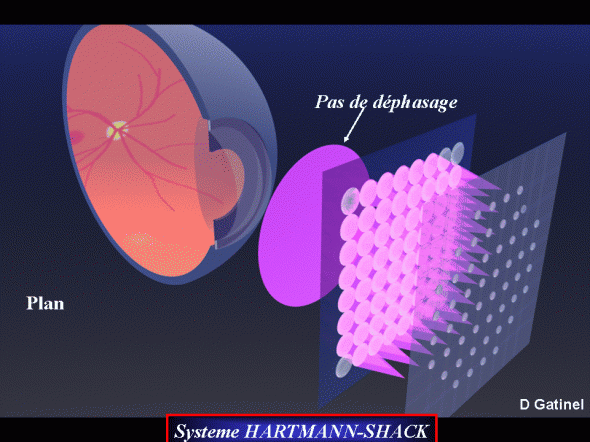No deviation is detected by Microlensing network, each portion of this "wave front" ideal to exit the eye being parallel to the plan of the Microlensing. There is no difference of optical path for all points of the pupil.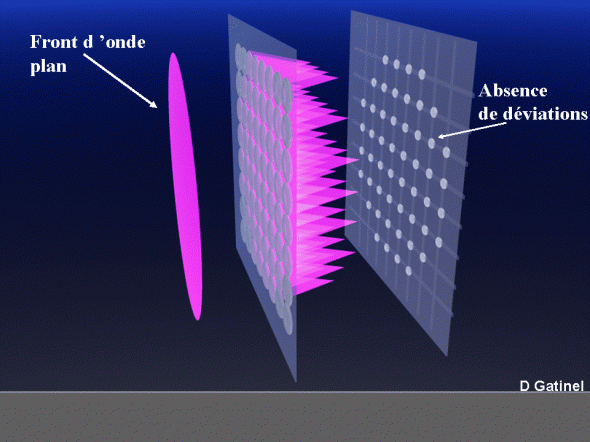If this same wave front was measured "inside" of the eye, it would be perfectly spherical and centered on the fovea.

### Study of the wave by retinal Imaging front

The study of the wavefront by retinal Imaging is uncommon in the clinic. It is based on the study of the deformation of a signal measured at the retinal level.
##### -Type Analyzer Tscherning
A network of thin light rays is obtained from a laser 532 nm wavelength filtering by a perforated mask. These rays are projected onto the retina through the 10 Central corneal mm, allowing obtaining a network of 13 × 13 luminous points distributed on a surface 1 Foveal mm square about. The image of this grid is collected by a CCD sensor and analyzed by comparison with the theoretical distribution obtained for an eye without optical aberrations.
##### -Launch of retinal rays: Tracey (retinal ray tracing) system
This system is based on a principle similar to that of type Tscherning Analyzer, but the distribution and collection of the image of the spots projected towards the retina is performed sequentially to avoid error of reconstruction, in particular for the study of the eyes with a high rate of optical aberrations (example: aberrometer iTrace, Tracey technologies).
##### -Adjustable incidental Refractometry (Ingoing adjustable refractometry)
It is based on the manual correction of the perception of light sources issued through a one-time location within the entrance pupil (principle of Scheiner disk). The degree of angulation for the superposition of the perceived light points measure the optical aberrations and is proportional to the slope of the wave front at the level of the region tested the entrance pupil.

### Study of the wave front by refractometry (OPD scan system) scanning

This system has a rotary transmitter device, which projects onto the retina a succession of trains of waves of infrared light of guidance different (Meridian by Meridian analysis) that are reflected on the retina, before be picked up out of the eyeball by a network of Photodetectors. When the eye is Emmetropic next to the Meridian analyzed, all of the photodecteurs is in turn simultaneously stimulated. In case of ametropia and/or optical aberrations, some photosensors are stimulated in early or late. The collection and study of the different temporal phase shifts allow the reconstruction of a Meridian by Meridian wave front. This system was developed by Nidek society.

### Analysis of the wave front

#### Representation of the wave front

The wave front is a theoretical construct that allows to represent the differences of perspective (or dephasing) path across the entrance pupil (in the case of the eye, the pupil of entry corresponds to the circumference of the IRIS Ward). To be intelligible, the wave front analysis requires that the aberrations that are there are characterized (qualified) and quantified (rate). The representation of the wave front can be performed by a decomposition into a sum of functions particularly interesting in this context: the Zernike polynomials. The coefficients which control have a value proportional to the optical aberration that corresponds to them. The first Zernike polynomials easily interpret, because they correspond to the phase shifts inflict optical aberrations 'classic' as the trefoil, the defocus, regular astigmatism, coma, etc.

The polynomials of Zernike database includes theoretically an infinite number of polynomials; However, the software that performs the decomposition of the wave front is working with a 'truncated base' (i.e. a limited number of polynomials). The number of chosen polynomials is low, more calculations will be fast, but approached (less accurate). In addition, the number of microlenses in the matrix of the aberrometric sensor sets the maximum number of sampling points involved in the calculation of decay of the wave front.

#### Principles of the decomposition in elementary signals

Analysis of the wave as a tridimensionelle surface front allows to understand the optical aberrations of the eye. The polynomials of Zernike decomposition allows to extract the useful information for the analysis of the wave front used; This is to convert the surface of the wave front in a sum of elementary areas, which correspond each to a degree and a particular type of optical aberration.
To understand this approach, just remember that any periodic signal, such acoustic as a note of music, can be broken down into a number of 'subsignals' basic (harmonics)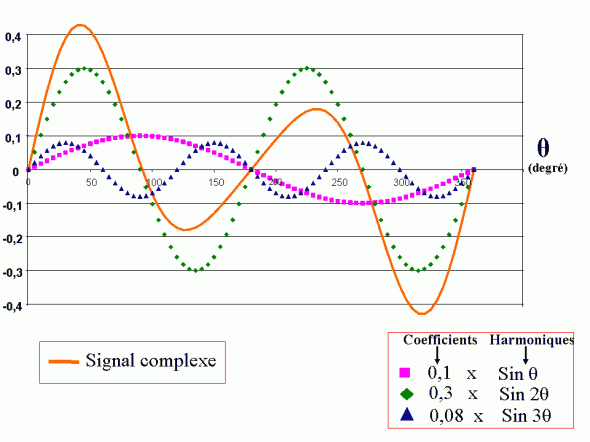Principle of the decomposition of a signal complex elementary signals. A periodic complex signal can be decomposed into elementary harmonic affected by a particular factor (spectral decomposition). In this example, three harmonics are sufficient to the decomposition of the original complex signal. The addition of each of these harmonics allows to reconstruct the complex signal: each harmonic is assigned a coefficient. It is high, and more influence in the form of the signal is important (in this example, the overall shape of the analyzed signal is close to the harmonic (Sin 2θ). This type of decomposition in sinusoidal function of increasing frequencies (2θ, θ, 3θ...) corresponds to the Fourier analysis, which aims to determine the value of the coefficients to be assigned to each of the harmonic in order to reconstruct the original signal by summation. In general, the signal is complex, and the more elementary signals necessary for its description. The principle used for the decomposition in polynomials of Zernike a surface such that the envelope of a wave front is similar. Each Zernike polynomial can be considered as a harmonic; the analysis of the wave front is by calculating the value of the coefficient assigned to each of the polynomials.

The transcript of a surface wave gathered in Zernike polynomials so akin to the decomposition of a note of music in harmonic signals. Depending on the type of instrument played, intensity (grade) of each harmonic will be different and an intensity value can be attributed to each harmonic signal. At the end of this process, it will be broken down the initial note in a sum of elementary signals assigned with a value of loudness (amplitude of the signal). Depending on the accuracy and the practical level of analysis, it is possible to reconstruct secondarily more or less faithfully the note played from the information provided by the initial work of decomposition. You can also extract the note one or more harmonics; a note can be "cleared" selectively unwanted harmonics (for a same note, the timbre of an instrument depends on the harmonic composition of the played note).

#### Interest of the Zernike polynomials for the description of the wave front

In aberrometr_e, the polynomials of Zernike particularly lend themselves to the decomposition of the wave front, share their mathematical properties and the fact that first polynomials to interpret as 'classic' optical aberrations in optics.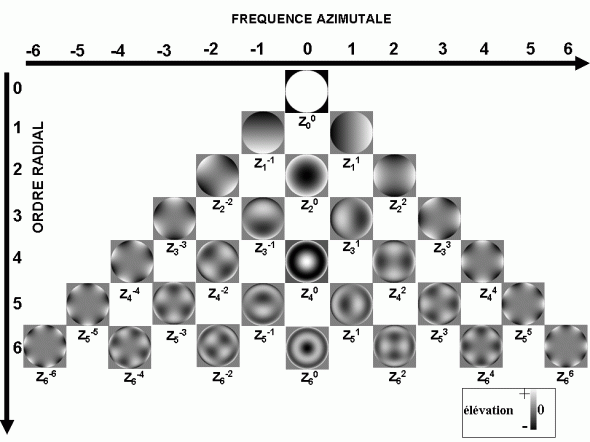Representation in gray scale corresponding to the vertical rise of the 28 first Zernike polynomials based on their frequency of Azimuthal and radial order.

-They are defined on a disk of unit RADIUS; However, the pupil through which the wave front is transmitted is similar also to a disc;
-the first Zernike polynomials have a practical physical interpretation, because as stated previously, they correspond pretty well to conventional optical aberrations;
-every polynomial can be assigned to a multiplicative digital coefficient; This happens when the decomposition of the surface of the front of waves by means of these polynomials;
-their mathematical expression is relatively simple, since they correspond to the product of a polynomial function with a trigonometric function; every polynomial family is defined by a degree or radial order (corresponding to the degree of the polynomial function) and a spatial frequency (for the trigonometric function);
-they have interesting mathematical properties: they are orthogonal each other, which makes it possible to selectively modify the coefficients without having to recalculate all of them. They are also standardized: the RMS value with the average plane is equal to 1 for each of the polynomials.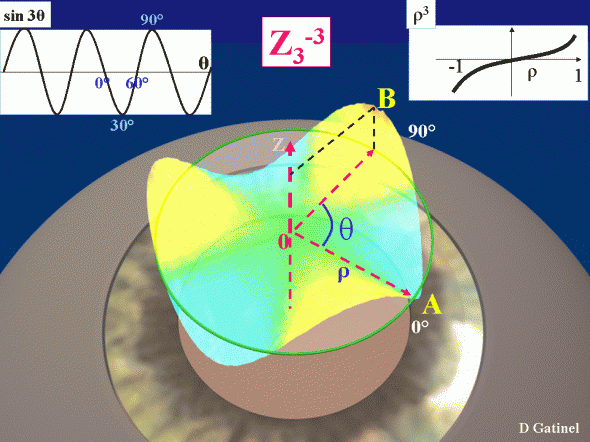Schematic representation of the Zernike polynomial (excluding normalization factor) corresponding to the triangular astigmatism (trefoil) Z3-3 on the pupillary standardized disk (RADIUS equal to 1) materialized on this figure by a green outline. It is equal to the product of a polynomial function of the radius of degree 3 (ρ3) where ρ represents the distance of the point considered in the Center, and a trig function of frequency Azimuthal equal to 3 (sin3θ), where θ is the angle made with the horizontal of the Meridian where the point is located.

The Zernike polynomials form a vector basis: they allow to mathematically describe any surface tridimensionelle to the circular edge by a set of coefficients, which each is assigned to a given polynomial. The sum of these polynomials affected their coefficients reproduced the surface analysis, and all the more faithfully than the number of analyzed points and polynomials used is important.

#### Decomposition of the front of waves in elementary polynomials

After measure deviations of the dot matrix compared to the reference grid (look ideal, devoid of optical aberrations), it is possible to reconstruct the wave like a slick formed portions front planes that are in each of the microlenses. Given the 'finished' numbers of these "facets" (equal number of microlenses), the surface is not continuous; a mathematical smoothing is therefore made, prior to the polynomials of Zernike decomposition. The number of polynomials that are assigned to this decomposition depends on the system used; a decomposition using the polynomials of degree less than or equal to 4 (which corresponds to 15 different polynomials) is the minimum sufficient decomposition for the analysis of the wave front collected.
The purpose of the decomposition is to determine the value of the coefficients for each of the polynomials. It is done through a complex matrix calculation made by computer, which is to minimize the difference between the expressed by the sum of the Zernike polynomials affected their coefficients and the surface returned by the parser of wave front.
The coefficients are determined so that the sum of the differences in elevation between the points of these high squared (Root Mean Square or RMS) surfaces is as low as possible. The polynomials of Zernike decomposition is only an approximation of the surface of the wave front, and may be subject to certain errors or inaccuracies, particularly for the fronts of waves with a high number of optical aberrations of high degree (patients operated of refractive surgery, Keratoconus, shift...) (4).
The mathematical decomposition of a wavefront in Zernike polynomials is a mathematical process that requires normalization of the radius of the pupil (the value of the radius of the pupil is assumed to be 1 for the calculation of the coefficients) . However, the distribution of polynomials and the value of each coefficient varies according to the pupillary diameter obtained during the collection of the wavefront. In other words, a variation in the pupillary diameter value of the same patient between two Tests induces a variation in the value of the coefficients attributed to the different aberrations present within the studied wavefront. In general, the larger the pupillary diameter, the higher the value of the coefficients assigned to the different polynomials
To be interpretable, the comparison between two successive reviews requires the collection or the calculation of the decomposition of the wave for a same pupillary diameter front.
In addition, the values assigned to each of the RMS coefficients of the polynomials used for the wavefront analysis should be interpreted with caution. While the quality of the visual function depends in part on the aberration rate within the wavefront, it is not directly proportional to this rate and the value of the coefficients considered separately or in a group. There are indeed compensations between the optical aberrations of different degrees derived from the decomposition in Zernike polynomials.

### Interpretation of the wave after polynomials of Zernike decomposition front

A resulting wave front of a theoretical look devoid of optical aberrations and collected by a system of type - Hartmann Schack is flat (neglecting the effects of diffraction that essentially would interest the frontd' wave collected through a pupil of small diameter). There is no optical phase shift, so no difference of optical path: the eye is optically 'perfect': only diffraction reduces the optical quality, and we speak of eye "limited by diffraction. Such an eye exists only in theory, because human eyes are always non-zero optical aberrations of high degree.
The existence of optical aberrations will induce a delay or a phase advance at certain points in the wave front. According to the degree of the optical aberration reporting, delays or advances of phase adopt a particular distribution. Certain optical aberrations are correlated with one of the first Zernike polynomials, and we will study them through each of these polynomials.

#### (A) Optical Aberrations based on their degree (radial order of classification of the Zernike polynomials)

Aberrations of degree 0
It's the "piston" type aberration: it is not responsible for optical aberration, because the wave front undergoes no deformation
Aberrations of degree 1
This is the tilt. They are the consequence of a failure of inclination of one or more elements constituting the optical system.
Aberrations of degree 2
This is the defocus, and astigmatism. These aberrations correspond to the Ametropias Sphéro-cylindrical axial (sphere and cylinder). The defocus corresponds to a parabolic deformation of the wavefront with rotational symmetry. Astigmatism also represents a parabolic deformation of the wavefront, but varies with the meridian in question and has axial symmetry. It corresponds to the combination of two saddle-shaped surfaces, the resultant of which is a surface of the same type but whose orientation provides the axis of astigmatism.
(see section astigmatism on the site)
Aberrations of degree 3
They correspond to the coma and trefoil-type aberrations ("Clover"). The polynomials expressing them do not show symmetry of rotation or axial symmetry. They reflect a lack of alignment (decentralization) of the elements constituting the optical system. Their rate increases significantly after LASIK or PKR, probably reflecting a relative imperfection in the centering of the treatment. They induce a predominantly asymmetrical phase shift on the "edges" of the wavefront (increasing with the distance in the centre of the pupil).
Aberrations of degree 4
These are the aberrations of sphericity. The polynomials expressing them all exhibit axial symmetry, and for some, rotational symmetry. They increase with the pupillary diameter and reflect a phase shift of the wavefront points located on the periphery of the pupil. They increase considerably after conventional LASIK and PKR, but also and in a lesser way after Aberrometry-guided surgery. They reflect the existence of suboptimal resurfacing at the periphery of the optical zone and/or an insufficient diameter of the latter relative to that of pupil.
Aberrations of degree higher than 4
Depending on the degree, they exhibit axial symmetry (even degrees) or not (odd degrees). They reflect the existence of unsystematized multiple optical imperfections that exert a particular effect on the edges of the wavefront.. They generally have a low impact on the visual function except when their rates are particularly high (such as during irregular corneal scarring, incisional surgery or Transfixiante keratoplasty).

#### (-B) Application to the interpretation of the wave front

Bear in mind that the "wave front" is an abstract representation of a phase for monochromatic light waves shift. The functions used for the mathematical representation of the wave front are same "abstract", but practice to quantify and characterize the optical defects of the eye.
The notion of 'phase' is more subtle than it seems. On a body of water which moves a train of ripples, water does not move horizontally, each molecule undergoes a vertical movement to the passage of the wave. The phase of these trains could be viewed as the trace relien the points on the surface of the water at the same height, and heading in the same direction (upwards or downwards). For a straight wave propagating 'straight', the phase will itself represented by a straight line.  A wave that'd to a point would have an outline of a circular stage. This wave would see its height increase as its spread (strengthening related to the convergence of the wave), to reach a centre where the amplitude of the vertical movement of water molecules located on this spot would be maximum. This centre would be, for light waves, the home. The photoreceptors are not sensitive to the phase (as all light detectors), but to the intensity (related to the amplitude of the wave).
The overall shape of a particular wave front depends on the optical aberrations that predominate in its genesis. Schematically:
-in case of important spherical ametropia, the wavefront will have a global parabolic shape! This parable can be approximated by a spherical couple in first approximation; It is centered on the punctum remotum of the Ametropia eye.
-In case of Ametropia myopia, the top of the parabola is directed backward (the center is retarded).
-In case of Ametropia Hypermétropique, the top is directed forward (the edges are retarded).
-the existence of an associated astigmatism mislead a slight axial asymmetry, particularly perceptible at the edges of the parable of revolution. In case of mixed astigmatism, the shape of the wavefront resembles that of a "Pringle" type of chips.
-The existence of a high spherical aberration rate induces elongation of the parabola, with depression or localized vaulted of its apex.
-Finally, the presence of coma-type aberrations induces an additional and asymmetric elongation of the parabola.
In the presence of a cylindrical sphéro ametropia, high-degree aberrations generally have a much lower rate than low-degree aberrations (tilt, defocus, astigmatism). The form of the phase-shift that they print is generally better analyzed when the low-degree aberrations of the wavefront representation are extracted.